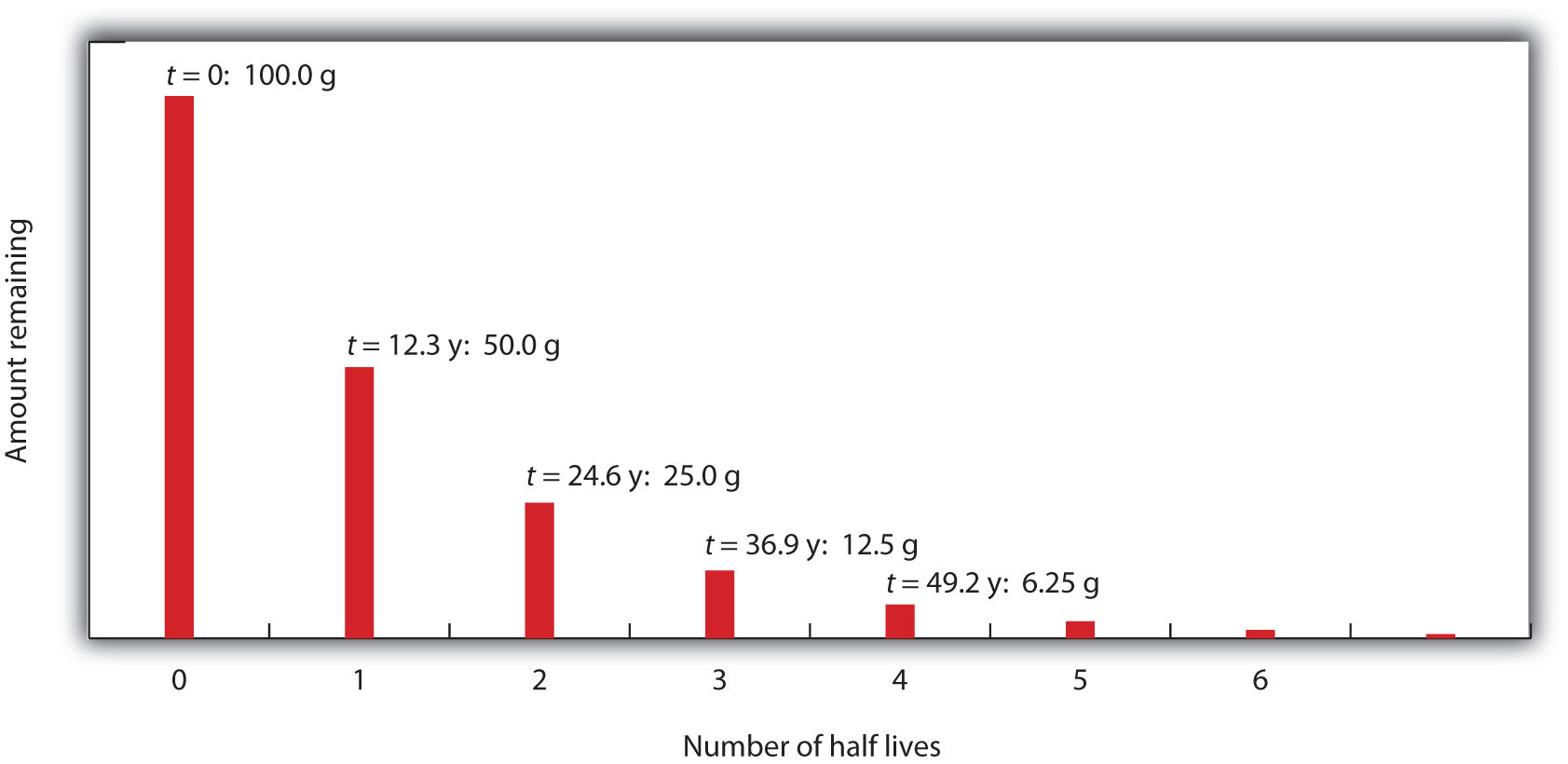This is “Half-Life”, section 11.2 from the book Introduction to Chemistry: General, Organic, and Biological (v. 1.0). For details on it (including licensing), click here.

Has this book helped you? Consider passing it on:
Creative Commons supports free culture from music to education. Their licenses helped make this book available to you.
DonorsChoose.org helps people like you help teachers fund their classroom projects, from art supplies to books to calculators.

## 11.2 Half-Life

### Learning Objective

1. Define half-life.
2. Determine the amount of radioactive substance remaining after a given number of half-lives.

Whether or not a given isotope is radioactive is a characteristic of that particular isotope. Some isotopes are stable indefinitely, while others are radioactive and decay through a characteristic form of emission. As time passes, less and less of the radioactive isotope will be present, and the level of radioactivity decreases. An interesting and useful aspect of radioactive decay is half-life. The half-lifeThe amount of time it takes for one-half of a radioactive isotope to decay. of a radioactive isotope is the amount of time it takes for one-half of the radioactive isotope to decay. The half-life of a specific radioactive isotope is constant; it is unaffected by conditions and is independent of the initial amount of that isotope.

Consider the following example. Suppose we have 100.0 g of 3H (tritium, a radioactive isotope of hydrogen). It has a half-life of 12.3 y. After 12.3 y, half of the sample will have decayed to 3He by emitting a beta particle, so that only 50.0 g of the original 3H remains. After another 12.3 y—making a total of 24.6 y—another half of the remaining 3H will have decayed, leaving 25.0 g of 3H. After another 12.3 y—now a total of 36.9 y—another half of the remaining 3H will have decayed, leaving 12.5 g of 3H. This sequence of events is illustrated in Figure 11.1 "Radioactive Decay".During each successive half-life, half of the initial amount will radioactively decay.

We can determine the amount of a radioactive isotope remaining after a given number half-lives by using the following expression:

where n is the number of half-lives. This expression works even if the number of half-lives is not a whole number.

### Example 3

The half-life of 20F is 11.0 s. If a sample initially contains 5.00 g of 20F, how much 20F remains after 44.0 s?

Solution

If we compare the time that has passed to the isotope’s half-life, we note that 44.0 s is exactly 4 half-lives, so using the previous equation, n = 4. Substituting and solving results in the following:

Less than one-third of a gram of 20F remains.

### Skill-Building Exercise

1. The half-life of 44Ti is 60.0 y. A sample initially contains 0.600 g of 44Ti. How much 44Ti remains after 180.0 y?

Half-lives of isotopes range from fractions of a microsecond to billions of years. Table 11.2 "Half-Lives of Various Isotopes" lists the half-lives of some isotopes.

Table 11.2 Half-Lives of Various Isotopes

Isotope Half-Life
3H 12.3 y
14C 5,730 y
40K 1.26 × 109 y
51Cr 27.70 d
90Sr 29.1 y
131I 8.04 d
222Rn 3.823 d
235U 7.04 × 108 y
238U 4.47 × 109 y
241Am 432.7 y
248Bk 23.7 h
260Sg 4 ms

### Looking Closer: Half-Lives of Radioactive Elements

Many people think that the half-life of a radioactive element represents the amount of time an element is radioactive. In fact, it is the time required for half—not all—of the element to decay radioactively. Occasionally, however, the daughter element is also radioactive, so its radioactivity must also be considered.

The expected working life of an ionization-type smoke detector (described in the opening essay) is about 10 years. In that time, americium-241, which has a half-life of about 432 y, loses less than 4% of its radioactivity. A half-life of 432 y may seem long to us, but it is not very long as half-lives go. Uranium-238, the most common isotope of uranium, has a half-life of about 4.5 × 109 y, while thorium-232 has a half-life of 14 × 109 y.

On the other hand, some nuclei have extremely short half-lives, presenting challenges to the scientists who study them. The longest-lived isotope of lawrencium, 262Lr, has a half-life of 3.6 h, while the shortest-lived isotope of lawrencium, 252Lr, has a half-life of 0.36 s. As of this writing, the largest atom ever detected has atomic number 118, mass number 293, and a half-life of 120 ns. Can you imagine how quickly an experiment must be done to determine the properties of elements that exist for so short a time?

### Concept Review Exercises

1. Define half-life.

2. Describe a way to determine the amount of radioactive isotope remaining after a given number of half-lives.

1. Half-life is the amount of time needed for half of a radioactive material to decay.

2. take half of the initial amount for each half-life of time elapsed

### Key Takeaways

• Natural radioactive processes are characterized by a half-life, the time it takes for half of the material to decay radioactively.
• The amount of material left over after a certain number of half-lives can be easily calculated.

### Exercises

1. Do all isotopes have a half-life? Explain.

2. Which is more radioactive—an isotope with a long half-life or an isotope with a short half-life?

3. How long does it take for 1.00 g of 103Pd to decay to 0.125 g if its half-life is 17.0 d?

4. How long does it take for 2.00 g of 94Nb to decay to 0.0625 g if its half-life is 20,000 y?

5. It took 75 y for 10.0 g of a radioactive isotope to decay to 1.25 g. What is the half-life of this isotope?

6. It took 49.2 s for 3.000 g of a radioactive isotope to decay to 0.1875 g. What is the half-life of this isotope?